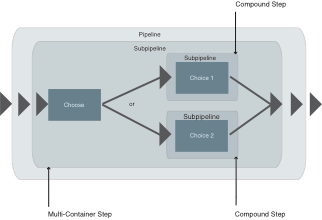XSL-FO - WordML - XSLT

# Multi-Container Steps

A Multi-Container Step has two or more alternative subpipelines. Thus it is possible amongst other things to realise implementations based on the distinction of cases.Figure: Multi-Container Steps in XProc

## The important Multi-Container Steps

There are two Multi-Container Steps in XProc: <p:choose> and <p:try>. In the following they are introduced and explained.

### p:choose

``````<p:choose name? = NCName>
(p:xpath-context?,
p:variable*,
p:when*,
p:otherwise?)
</p:choose>``````

With <p:choose> case-related choice-making processes can be implemented. On the basis of an appropriate XSLT expression defined in <p:when>, the corresponding subpipelines are carried out depending on the result. If no condition of <p:when> applies, <p:otherwise> (if indicated) will be carried out. This corresponds to a default value.

``````<p:when test = XPathExpression>
(p:xpath-context?,
(p:output |
p:log)*,
subpipeline)
</p:when>``````

A XPath expression has been deposited as attribute under "test" by the <p:when> step. If it applies, its subpipeline will be carried out. In addition, the step can also be configured and extended by <p:xpath-context>, <p:log> and <p:output>.

``````<p:otherwise>
((p:output |
p:log)*,
subpipeline)
</p:otherwise>``````

<p:otherwise> is the standard way followed by <p:choose> in the event that all query conditions are not met. Depending on the choice, the corresponding subpipeline will be carried out and the result will be written on the ouput port.

#### Example

In the following example the <p:choose> step is introduced.

``````<?xml version="1.0" encoding="UTF-8"?>
<p:declare-step xmlns:p="http://www.w3.org/ns/xproc" xmlns:c="http://www.w3.org/ns/xproc-step" version="1.0">
<p:input port="source">
<p:document href="FilmCollection.xml"/>
</p:input>
<p:output port="result">
<p:empty/>
</p:output>
<p:choose>
<p:when test="count(//Film) &gt; 1">
<p:store href="morethanonefile.xml"/>
</p:when>
<p:when test="count(//Film) = 1">
<p:store href="onefile.xml"/>
</p:when>
<p:otherwise>
<p:store href="standard.xml"/>
</p:otherwise>
</p:choose>
</p:declare-step>``````

In this example the corresponding XPath expressions within <p:when> are testing how many “Film“ elements are available in the initial document. According to the case, an appropriate file will be generated.

### p:try

``````<p:try name? = NCName>
(p:variable*,
p:group,
p:catch)
</p:try>``````

Within a <p:try> step any dynamic errors can be intercepted prior to an interruption. The desired subpipeline is compounded and carried out within a <p:group> step. If an error occurs, it will be cought by a so-called <p:catch> step.

``````<p:catch name? = NCName>
((p:output |
p:log)*,
subpipeline)
</p:catch>``````

Instead of an interruption, a subpipeline will be carried out within the <p:catch> step. Depending on the situation, this can be an accordingly formulated error output or an alternative way with further steps.

#### Example

In the following example an error is deliberately produced in order to enter the subpipeline of <p:catch>.

``````<?xml version="1.0" encoding="UTF-8"?>
<p:declare-step xmlns:p="http://www.w3.org/ns/xproc" xmlns:c="http://www.w3.org/ns/xproc-step" version="1.0">
<p:input port="source">
<p:document href="FilmCollection.xml"/>
</p:input>
<p:output port="result"/>
<p:try>
<p:group>
<p:validate-with-xml-schema assert-valid="true" mode="strict">
<p:input port="schema">
<p:document href="example_5_xmlschema.xsd"/>
</p:input>
</p:validate-with-xml-schema>
</p:group>
<p:catch>
<p:identity>
<p:input port="source">
<p:inline>
<c:result>Unfortunately, the document is not valid.</c:result>
</p:inline>
</p:input>
</p:identity>
</p:catch>
</p:try>
</p:declare-step>``````

If the <p:validate-with-xml-schema> step produces an error, so that the initial document is not valid against the underlying Schema file, the <p:catch> step will be called up and its subpipeline will be outputted. In this example it is a <p:inline> output with an explanatory text. So, if the document is not valid, "Unfortunately, the document is not valid." will be outputted.